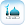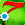# SUMOO, Multiplayer Math Puzzle

by Masarat App
10,000+ installs

Android application SUMOO, Multiplayer Math Puzzle developed by is listed under category Game Puzzle. According to Google Play SUMOO, Multiplayer Math Puzzle achieved more than 10,000 installs. SUMOO, Multiplayer Math Puzzle currently has 550 ratings with average rating value of 4.6. The current percentage of ratings achieved in last 30 days is 0.04%, percentage of ratings achieved in last 60 days is 0.08%. SUMOO, Multiplayer Math Puzzle has the current market position #164305 by number of ratings. A sample of the market history data for SUMOO, Multiplayer Math Puzzle can be found below. Last update on 2023-09-02.

Title: SUMOO, Multiplayer Math Puzzle Masarat App Game Puzzle Free Android
Total ratings: 550 0.04% 0.08% 4.6
Installs (achieved): 10,000+ 27,093
 5 star ratings: 488 0 0 30 30

2016-06-18: Android application SUMOO, Multiplayer Math Puzzle achieved 10,000 installs. Android application SUMOO, Multiplayer Math Puzzle achieved 5,000 installs. Android application SUMOO, Multiplayer Math Puzzle achieved 1,000 installs.

Total number of ratings
Total number of active users rated for SUMOO, Multiplayer Math Puzzle.

Total number of installs (*estimated)
Estimation of total number of installs on Google Play. Approximated from number of ratings and install bounds achieved on Google Play.

Average rating
Average rating value on Google Play. Given by active users of the application.